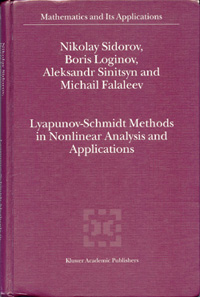# SIDOROV N., LOGINOV B., SINITSYN A., FALALEEV M. Lyapunov-Schmidt Methods in Nonlinear Analysis and ApplicationsSidorov N., Loginov B., Sinitsyn A., Falaleev M. Lyapunov–Schmidt Methods in Nonlinear Analysis and Applications. — Dordrecht–Boston–London: Kluwer Academic Publisher, 2002. — 568 p.This book concentrates on the branching solutions of nonlinear operator equations and the theory of degenerate operator-differential equations especially applicable to algorithmic analysis and nonlinear PDE's in mechanics and mathematical physics.The authors expound the recent result on the generalized eigen-value problem, the perturbation method, Schmidt's pseudo-inversion for regularization of linear and nonlinear problems in the branching theory and group methods in bifurcation theory.The book covers regular iterative methods in a neighborhood of branch points and the theory of differential-operator equations with a non-invertible operator in the main expression is constructed. Various recent results on theorems of existence are given including asymptotic, approximate and group methods. The reduction of some mathematics, physics and mechanics problems (capillary-gravity surface wave theory, phase transitions theory, Andronov-Hopf bifurcation, boundary-value problems for the Vlasov-Maxwell system, filtration, magnetic insulation) to operator equations gives rich opportunities for creation and application of stated common methods for which existence theorems and the bifurcation of solutions for these applications are investigated.Audience The book will be of interest to mathematicians, mechanics, physicists and engineers interested in nonlinear equations and applications to nonlinear and singular-systems as well as to researchers and students of these topics.